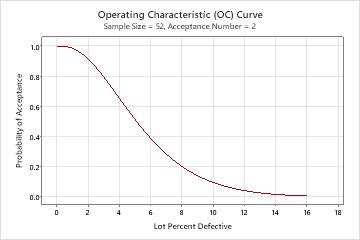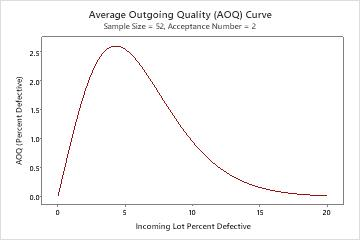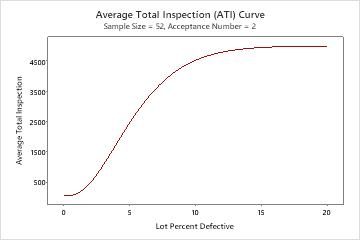# All statistics and graphs for Attributes Acceptance Sampling

Find definitions and interpretation guidance for every statistic and graph that is provided with an attributes acceptance sampling plan.

## Measurement type

For an attributes sampling plan, you can count the number of defectives in your sample (go/no go data), or you can count the number of defects.
Defect
A defect is a fault in a single item, such as a stain on a shirt. An item can have more than one defect.
Defective
A defective is a nonconforming item, such as a pen that does not work. An item is either defective or not defective.

## Lot quality

The lot quality units of measure for an attributes acceptance sampling plan depend on whether you choose to count defective items or defects.

If you count defective items, the following are units of measure that you can use.
Percent defective
Represents the percentage defective as a value between 0 and 100. For example, if 10 units are defective out of a sample size of 500, the percent defective is 2.
Proportion defective
Represents the proportion defective as a value between 0 and 1. For example, if 10 units are defective out of a sample size of 500, the proportion defective is 0.02.
Defectives per million
Represents the level of defectives as a value out of a million units. For example, 10 defectives per million (DPM) means that you have 10 defective units for every million units.
If you count defects, the following are units of measure that you can use.
Defects per unit
Defects per unit (DPU) is the average number of defects per unit observed when sampling a population.
Defects per hundred
Defects per hundred (DPH) is the average number of defects per hundred units observed when sampling a population.
Defects per million
Defects per million (DPM) is the average number of defects per million units observed when sampling a population.

## Lot size

The lot size is the population that you collect your samples from when you decide whether to accept or reject the entire lot.

Often, the lot size is chosen to be convenient for shipping and handling for both the supplier and consumers. For example, a convenient lot size might be an entire shipment. Because sampling plans assume homogeneity of parts in a lot, the units that comprise a lot should be produced under the same process conditions. Also, larger lots are generally more economical to inspect than a series of smaller lots.

## Acceptable Quality Level (AQL) and Rejectable Quality Level (RQL or LTPD)

Acceptable quality level (AQL)
The acceptable quality level (AQL) is the highest defective rate or defect rate from a supplier's process that is considered acceptable. The AQL describes what the sampling plan will accept, and the RQL describes what the sampling plan will reject. You want to design a sampling plan that accepts a particular lot of product at the AQL most of the time.
For example, you receive a shipment of microchips, and the AQL is 1.5%. Because you know that you won't always make the correct decision (sampling risk), you set the producer's risk (α) = 0.05. This means that approximately 95% of the time, you will correctly accept a lot with a defective rate of 1.5% or less, and 5% of the time, you will incorrectly reject the lot with a defective rate of 1.5% or less.
Rejectable quality level (RQL or LTPD)
The rejectable quality level (RQL) is the highest defective rate or defect rate that the consumer is willing to tolerate in an individual lot. The RQL describes what the sampling plan will reject, and the AQL describes what the sampling plan will accept. You want to design a sampling plan that rejects a particular lot of product at the RQL most of the time.
For example, you receive a shipment of microchips and the RQL is 6.5%. Because you know that you won't always make the correct decision (sampling risk), you set the consumer's risk (β) = 0.10. This means that, at least 90% of the time, you will reject a lot with a defective rate of 6.5% or more. At most 10% of the time, you will accept the lot with a defective rate of 6.5% or more.

### Interpretation

The consumer and supplier should agree to the highest defective rate or defect rate that is acceptable (AQL). The consumer and supplier should also agree to the highest defective rate or defect rate that the consumer will tolerate in an individual lot (RQL).

The probability of acceptance at the AQL (1.5% defective) is 0.95, and the probability of rejecting is 0.05. The probability of accepting at the RQL (10% defective) is 0.10, and the probability of rejecting is 0.90.
Method Acceptable Quality Level (AQL) 1.5 Producer’s Risk (α) 0.05 Rejectable Quality Level (RQL or LTPD) 10 Consumer’s Risk (β) 0.1

## Producer's risk (Alpha) and consumer's risk (Beta)

In acceptance sampling, you decide to accept or reject an entire lot based on the results from inspecting a sample from that lot. Because your decision to reject or accept the lot is based only on a sample, not on data from the entire lot, you risk rejecting good lots (producer's risk) and accepting bad lots (consumer's risk).
Producer’s risk (Alpha)
The producer's risk, α, is the probability of rejecting a lot that has a quality level equal to the AQL that should be accepted. As α increases, the risk of rejecting lots with defective rates equal to the AQL increases, which causes harm to the producer. The producer's risk is also known as type I error.
1 – α represents the desired probability of accepting a lot at the AQL.
Consumer’s risk (Beta)
The consumer's risk, β, is the probability of accepting a lot with a quality level equal to the RQL that should be rejected. As β increases, the risk of accepting lots with defective rates equal to the RQL increases, which causes harm to the consumer. The consumer's risk is also known as type II error.
β represents the desired probability of accepting a lot at the RQL.

### Interpretation

To protect the producer, the risk of rejecting a lot that has acceptable quality must be low. To protect the consumer, the risk of accepting a lot that has poor quality must be low.

The probability of acceptance at the AQL is 0.95, and the probability of rejecting is 0.05. The probability of accepting at the RQL is 0.10, and the probability of rejecting is 0.90.
Method Acceptable Quality Level (AQL) 1.5 Producer’s Risk (α) 0.05 Rejectable Quality Level (RQL or LTPD) 10 Consumer’s Risk (β) 0.1

## Sample size

In acceptance sampling, the sample size is the number of items that are randomly chosen from a single lot for inspection.

### Interpretation

In this example, the sample size is 52. You must sample 52 items from the entire lot of product.
Generated Plan(s) Sample Size 52 Acceptance Number 2 Accept lot if defective items in 52 sampled ≤ 2; Otherwise reject.

## Acceptance number

The acceptance number is the maximum number of defects or defectives that are allowed in a sample from an acceptable lot.

### Interpretation

In this example, the acceptance number is 2. You must sample 52 items from the entire lot of product. If 2 or less defective items are found, you accept the entire lot. If 3 or more defective items are found, you reject the entire lot.
Generated Plan(s) Sample Size 52 Acceptance Number 2 Accept lot if defective items in 52 sampled ≤ 2; Otherwise reject.

## Probability accepting and probability rejecting

The probability of accepting lots at the AQL should be close to 1 – α. The probability of accepting lots at the RQL should be close to β. The probability of rejecting is 1 – the probability of accepting.

### Interpretation

The probability of acceptance at the AQL (1.5% defective) is 0.957, and the probability of rejecting is 0.043. The probability of accepting at the RQL (10.0% defective) is 0.097, and the probability of rejecting is 0.903.
Percent Probability Probability Defective Accepting Rejecting AOQ ATI 1.5 0.957 0.043 1.420 266.2 10.0 0.097 0.903 0.956 4521.9

## AOQ and AOQL

The average outgoing quality level represents the relationship between the quality of the incoming material and the quality of the outgoing material, assuming that rejected lots will be 100% inspected and all defective items will be replaced or reworked.

When the incoming quality is very good or very bad, the outgoing quality is usually good because of the sorting and reworking of the bad parts so they don't get through the process. When the incoming quality is mediocre, the outgoing quality gets worse and the % defective reaches a maximum known as the average outgoing quality limit (AOQL).
###### Note

You must specify the lot size in order to calculate the AOQ and AOQL.

### Interpretation

In this example, when the average incoming quality level is 1.5% defective, the average outgoing quality is 1.42% defective. When the average incoming quality level is 10.0% defective, the average outgoing quality is 0.956% defective. The incoming quality is worse than the outgoing quality because rejected lots will be 100% inspected and will have all nonconforming units replaced or reworked.

The worst average outgoing defect level (AOQL) of 2.603% defective occurs when the incoming quality level is 4.3% defective.

### Acceptance Sampling by Attributes

Measurement type: Go/no go Lot quality in percent defective Lot size: 5000 Use binomial distribution to calculate probability of acceptance
Method Acceptable Quality Level (AQL) 1.5 Producer’s Risk (α) 0.05 Rejectable Quality Level (RQL or LTPD) 10 Consumer’s Risk (β) 0.1
Generated Plan(s) Sample Size 52 Acceptance Number 2 Accept lot if defective items in 52 sampled ≤ 2; Otherwise reject.
Percent Probability Probability Defective Accepting Rejecting AOQ ATI 1.5 0.957 0.043 1.420 266.2 10.0 0.097 0.903 0.956 4521.9
Average Outgoing Quality Limit(s) (AOQL) At Percent AOQL Defective 2.603 4.300

## ATI

The average total inspection (ATI) per lot represents the relationship between the quality of the incoming material and the average number of items that need to be inspected, assuming that rejected lots will be 100% inspected.
###### Note

You must specify the lot size in order to calculate the ATI.

### Interpretation

In this example, when the average incoming quality level is 1.5% defective, the average number of units inspected per lot is 266.2. This is because 95.7% of the time you will inspect 52 items and pass the lot, and 4.3% of the time you will reject the lot and inspect all 5000 items. When the average incoming quality level is 10.0% defective, the average number of units inspected per lot is 4521.9, which is almost the entire shipment.
Percent Probability Probability Defective Accepting Rejecting AOQ ATI 1.5 0.957 0.043 1.420 266.2 10.0 0.097 0.903 0.956 4521.9

## OC curve

The operating characteristic (OC) curve shows the ability of an acceptance sampling plan to distinguish between good and bad quality lots. The OC curve plots the probability of accepting lots that have different incoming quality levels for each sampling plan.

### Interpretation

In this example, if the actual % defective is 1.5%, you have a 0.957 probability of accepting this lot based on the sample and a 0.043 probability of rejecting it. If the actual % defective is 10%, you have a 0.097 probability of accepting this lot and a 0.903 probability of rejecting it.## AOQ curve

The average outgoing quality (AOQ) curve shows the relationship between the quality of the incoming material and the quality of the outgoing material, assuming that rejected lots will be 100% inspected and defective items will be replaced or reworked and inspected again (rectifying inspection).
###### Note

You must specify the lot size in order to create an AOQ curve.

### Interpretation

In this example, when the average incoming quality level is 1.5% defective, the average outgoing quality is 1.42% defective. When the average incoming quality level is 10.0% defective, the average outgoing quality is 0.956% defective. The incoming quality is worse than the outgoing quality because rejected lots will be 100% inspected and will have all nonconforming units replaced or reworked.

The worst average outgoing defect level (AOQL) of 2.603% defective occurs when the incoming quality level is 4.3% defective.## ATI curve

The average total inspection (ATI) curve shows the relationship between the quality of the incoming material and the number of items that need to be inspected, assuming that rejected lots will be 100% inspected and defective items will be replaced or reworked and inspected again (rectifying inspection).
###### Note

You must specify the lot size in order to create an ATI curve.

### Interpretation

In this example, when the average incoming quality level is 1.5% defective, the average number of units inspected per lot is 266.2. This is because 95.7% of the time you will inspect 52 items and pass the lot, and 4.3% of the time you will reject the lot and inspect all 5000 items. When the average incoming quality level is 10.0% defective, the average number of units inspected per lot is 4521.9, which is almost the entire shipment.By using this site you agree to the use of cookies for analytics and personalized content.  Read our policy